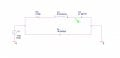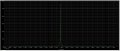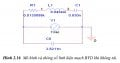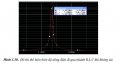# Problem in RLC Resonator, VAC source is 1V but FFT graph show ~ KV

#### Ngô Đình Nhân

Joined Jan 8, 2017
45
Hi all
I am trying to obtain a resonance frequency of an RLC circuit by using Pspice 9.2.
Simulation result turned to the proper resonance frequency, however, there may have a problem in the amplitude of the signal. I am using VAC = 1V but in FFT graph, the amplitude was in a range of ~ kV.

TIA#### crutschow

Joined Mar 14, 2008
27,956
Yes, I measured about a 1.7kV peak in my LTspice simulation of that circuit also.
It's due to the large inductance and very small capacitance in series (is ≈48fF the correct value?).
It practice the parasitic circuit capacitances would likely not allow the voltage to get anywhere near that high a value.

#### kubeek

Joined Sep 20, 2005
5,765
In practice the inductor will have quite large parastic capacitance parallel to it, and the self-resonance of the inductor will me somwhere in the hundreds of kHz most likely.

#### Bordodynov

Joined May 20, 2015
2,916
There is no real voltage of this magnitude. It is only in an equivalent circuit that such a voltage acts.

#### Ngô Đình Nhân

Joined Jan 8, 2017
45

#### DickCappels

Joined Aug 21, 2008
7,905
Cp can be ignored since it is not in the circuit -both ends are grounded.

Yes, it is real voltage but you can't get much current at that input to output voltage ratio. It is a straight-forward series tuned resonant circuit. At resonance the current is limited by the 1 volt source divided by Rm. Multiply that current by the reactance of Cm. If all components were ideal the output voltage would be infinite, but luckily, real parts are not idea so we don't have infinite voltages in our world.

Note that you can consider that the inductor to be a current source and that means that any load put on the output will reduce the voltage.

It is a valid power supply topology in which the output voltage can be adjusted by controlling the drive frequency or the resonant frequency (as with saturable inductors).

http://iopscience.iop.org/article/10.1088/1742-6596/418/1/012061/pdf

•kubeek

#### Ngô Đình Nhân

Joined Jan 8, 2017
45

but actually, I want to model a Quartz crystal in Pspice, so its equivalent circuit is RLC resonator. That means I can not remove or add any component to this circuit.
So I am considering that why that author can simulate that RLC circuit with a real voltage without add or remove anything. Is there any way of modifying each of component R, L, C to make them more realistic?#### Bordodynov

Joined May 20, 2015
2,916
I counted the quartz generator many times. I was calculating real circuits (I was a former developer of microcircuits for quartz clocks) and after seeing your circuit, I immediately realized that you are trying to simulate a quartz crystal. Read again my remark. I can add that the real value in this equivalent circuit is the current (inductance and capacitance), the voltages are only theoretical values. This real current causes small voltages (comparatively) in the external circuit. These quantities symbolize the mechanical stresses in a quartz crystal. If you search, you can find ready-made models of quartz resonators on the Internet. In Pspice (ORCAD) they also are.

•Danko

#### Ngô Đình Nhân

Joined Jan 8, 2017
45
Hello Mr.Bordodynov

Actually, I am designing an oscillator circuit based on Quartz, then I need to model quartz in PSpice and also need to obtain a real value of voltage. This is important because I need to calculate the value of other components in my circuit.
Do you have any idea for my case?

#### kubeek

Joined Sep 20, 2005
5,765
... Actually, I am designing an oscillator circuit based on Quartz, then I need to model quartz in PSpice and also need to obtain a real value of voltage. This is important because I need to calculate the value of other components in my circuit....
Why do you need the "voltage" inside the crystal, when this voltage is a model of some completely different physical quantity (probably strain)?
Outside of that model the voltage and current will represent the actual voltage and current of a crystal, but don´t bother measuring anything inside the model.